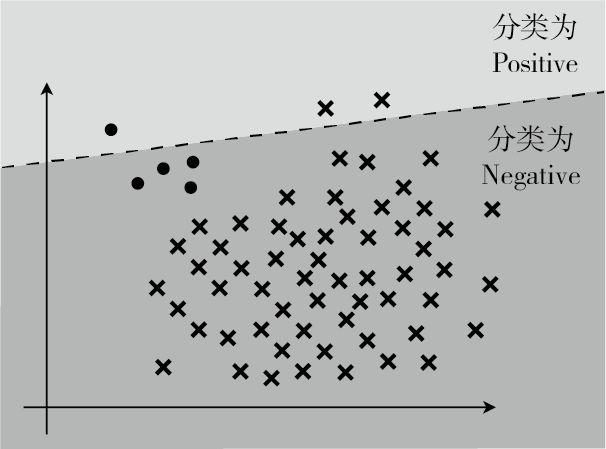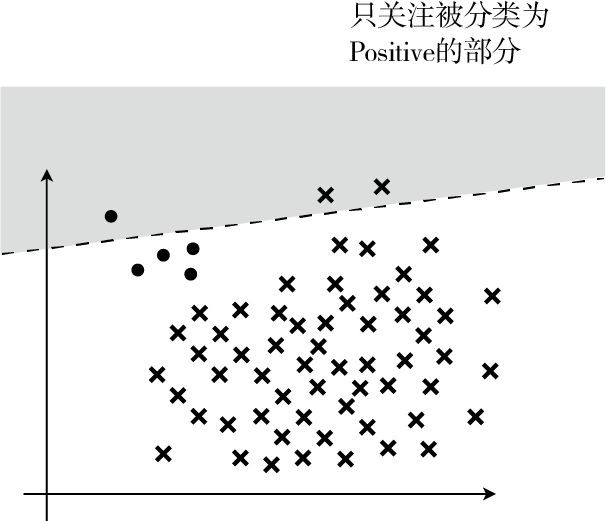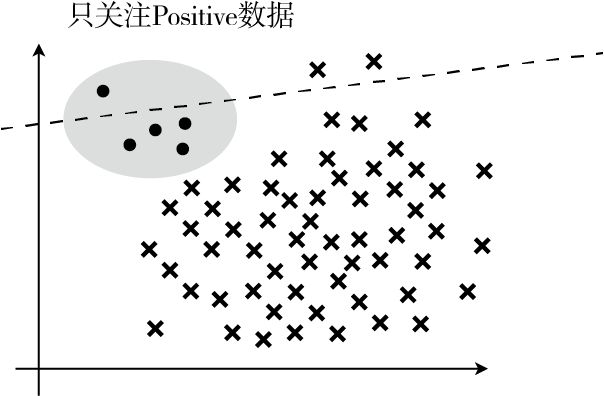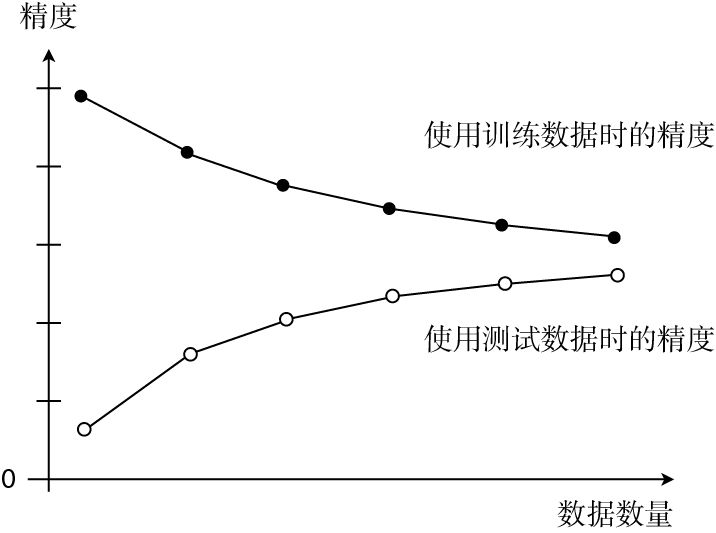# 知识点梳理

## 梯度下降法

$$x := x - \eta \frac{\text{d}}{\text{d}x}g(x) \tag{1}$$

A := B 这种写法，它的意思是通过 B 来定义 A。拿表达式（1）来说就是用上一个 $x$ 来定义新的 $x$。 $\eta$ 是称为学习率的正的常数，读作「伊塔」。根据学习率的大小，到达最小值的更新次数也会发生变化。换种说法就是收敛速度会不同。有时候甚至会出现完全无法收敛，一直发散的情况。

## 分类$$Fmeasure = \frac{2}{\frac{1}{Precision} + \frac{1}{Recall}} = \frac{2 \cdot Precision \cdot Recal}{Precision + Recall} \tag{2}$$

## 交叉验证

1. 把全部训练数据分为 $K$ 份；
2. 将 $K-1$ 份数据用作训练数据，剩下的 1 份用作测试数据；
3. 每次更换训练数据和测试数据，重复进行$K$ 次交叉验证；
4. 最后计算 $K$ 个精度的平均值，把它作为最终的精度。

## 过拟合

1. 增加全部训练数据的数量
2. 使用简单的模型
3. 正则化

$\theta_{0}$ 这种只有参数的项称为偏置项，一般不对它进行正则化。 $\lambda$ 是决定正则化项影响程度的正的常数。这个值需要我们自己来定。

## 学习曲线